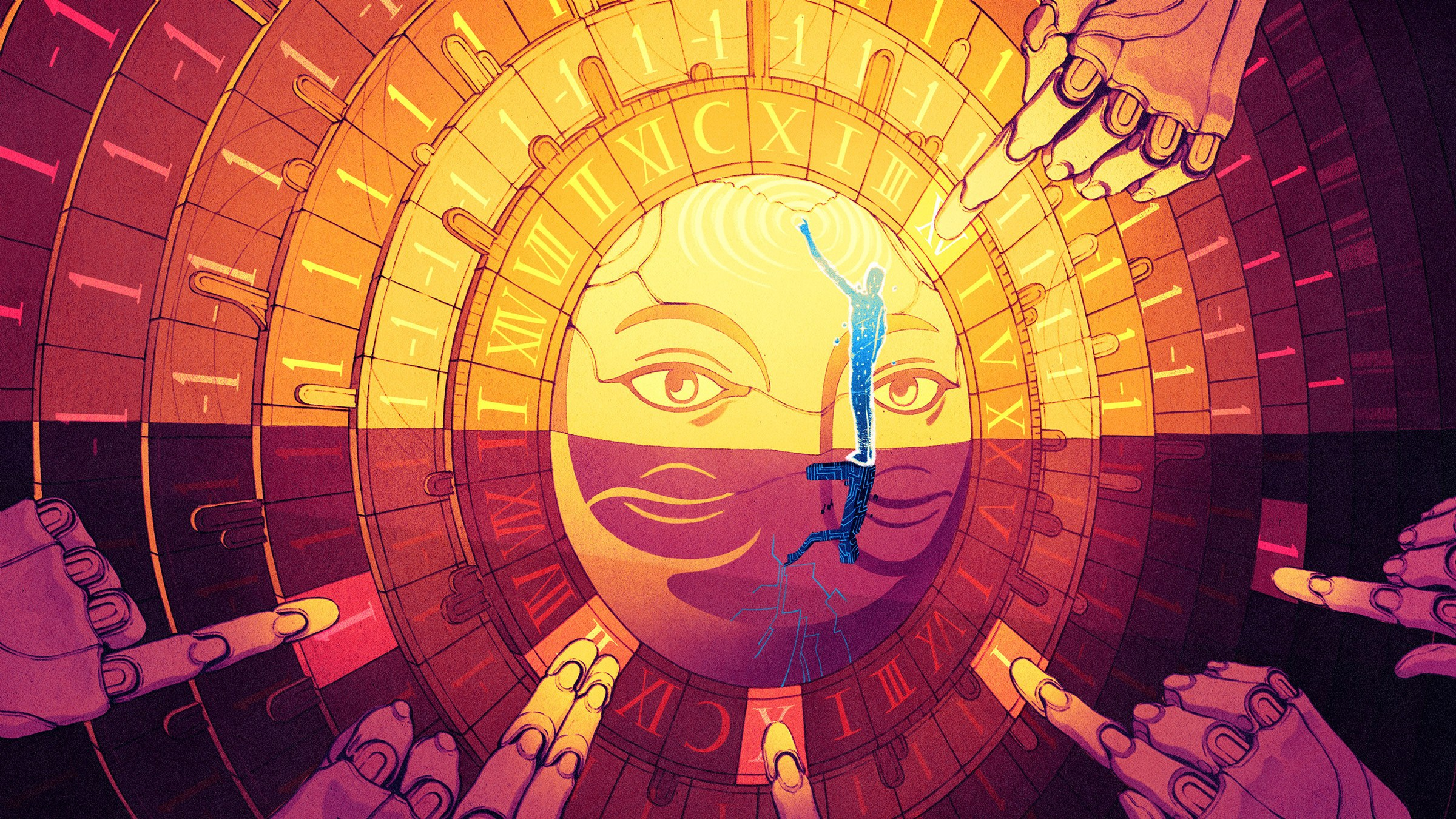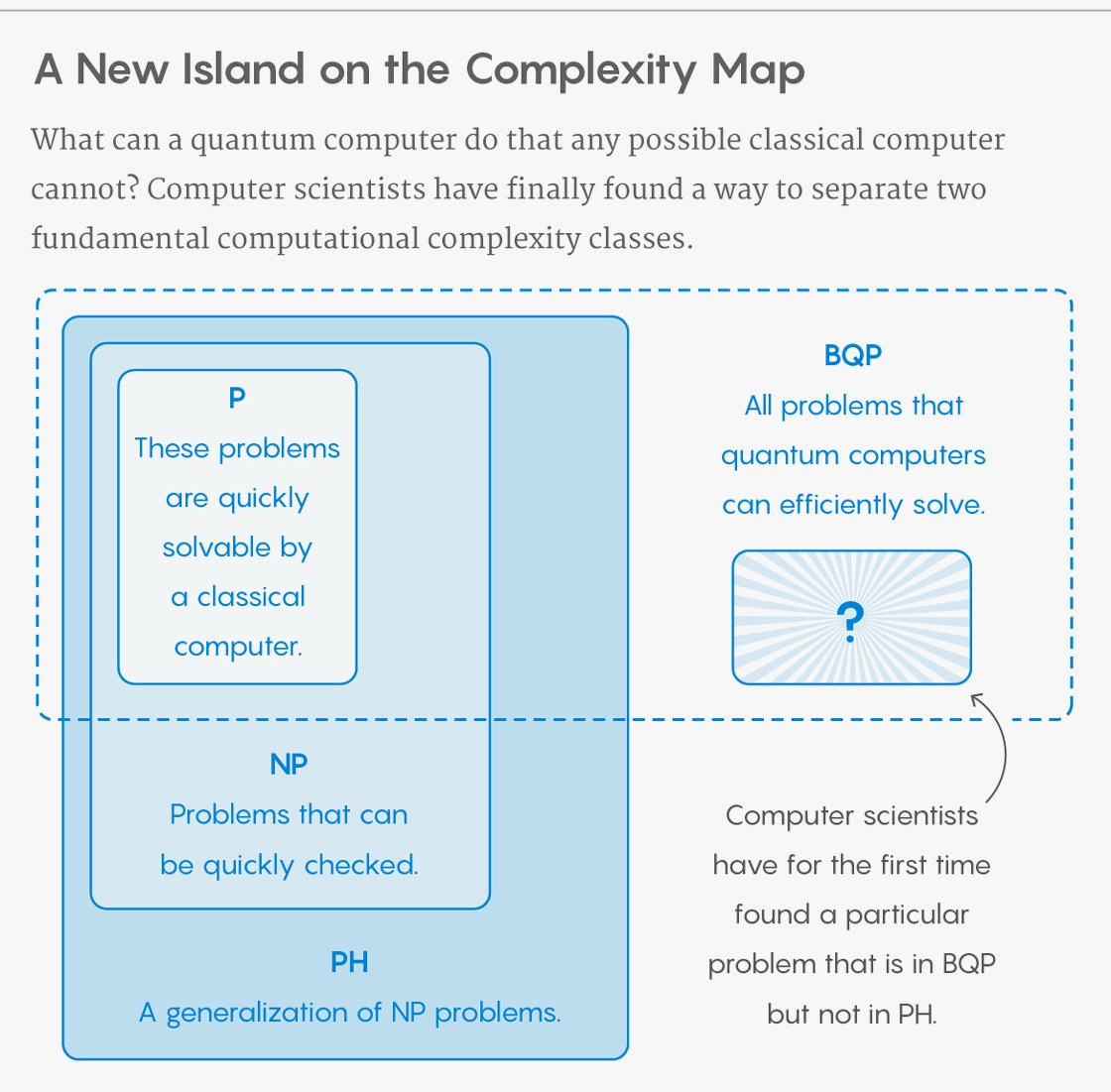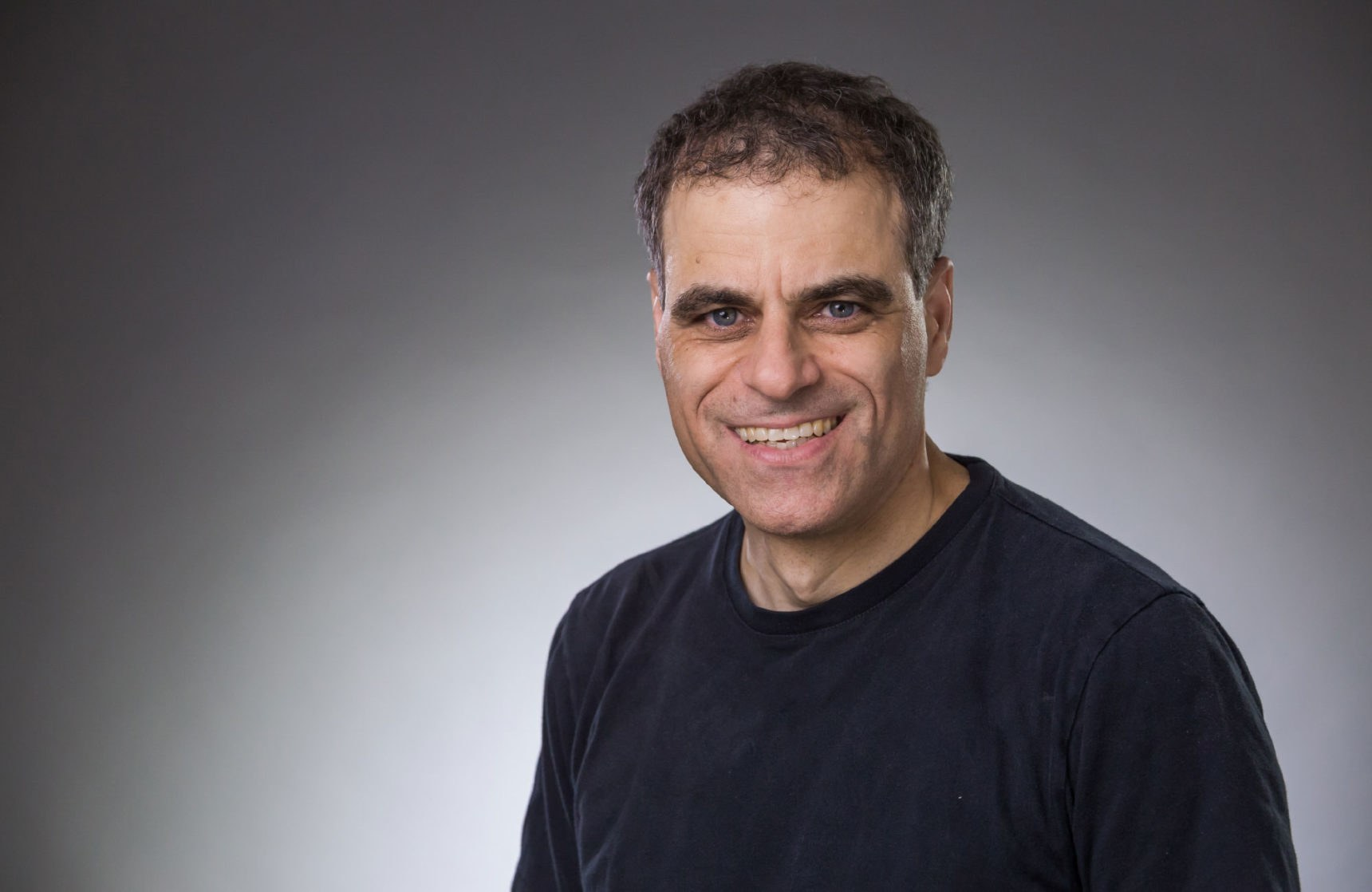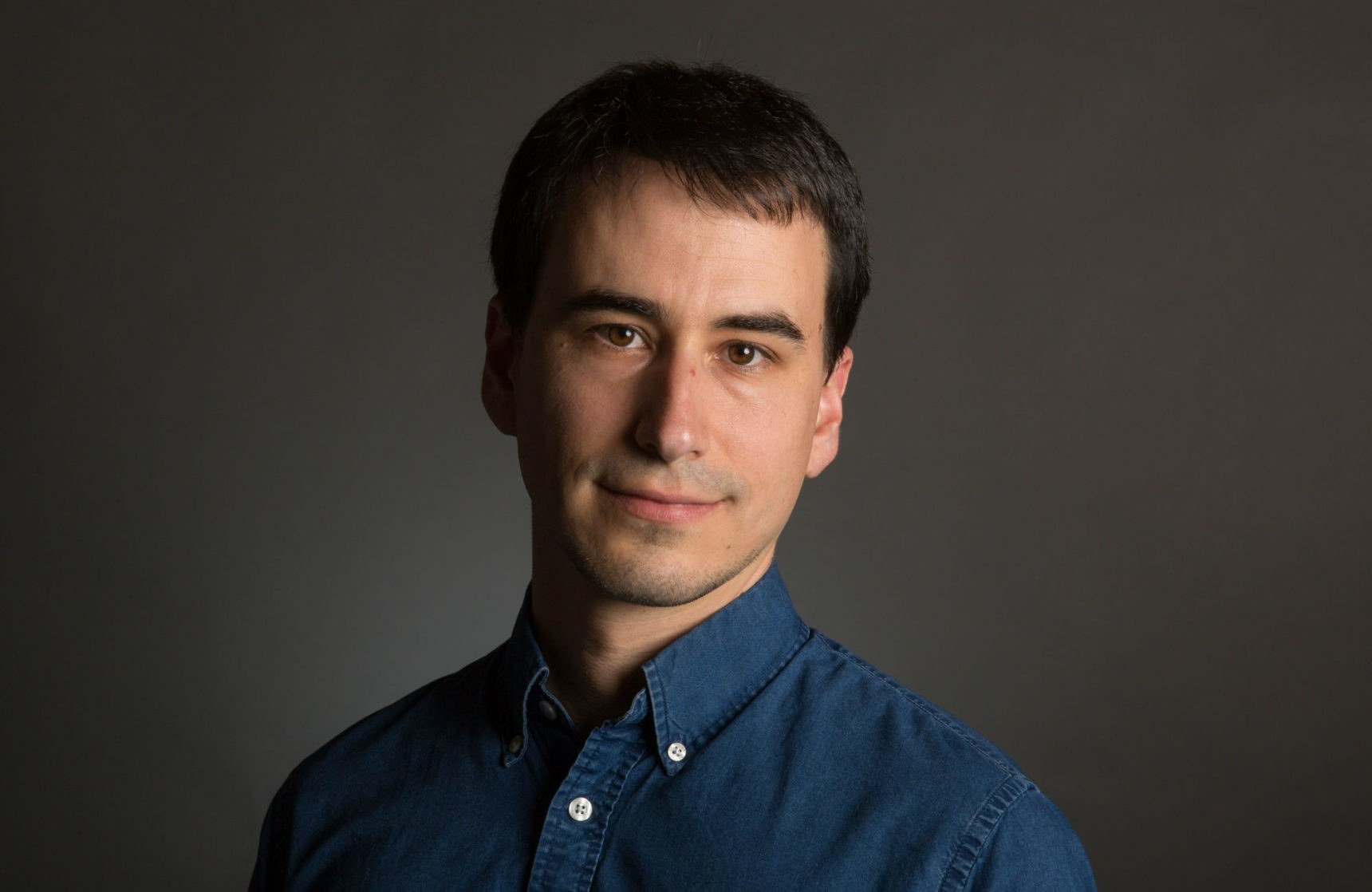# Finally, A Problem Only Quantum Computers Will Ever Be Able to Solve

Wired • TAGS:Kevin Hong for Quanta Magazine

Early on in the study of quantum computers, computer scientists posed a question whose answer, they knew, would reveal something deep about the power of these futuristic machines. Twenty-five years later, it’s been all but solved. In a paper posted online at the end of May, computer scientists Ran Raz and Avishay Tal provide strong evidence that quantum computers possess a computing capacity beyond anything classical computers could ever achieve.

Raz, a professor at Princeton University and the Weizmann Institute of Science, and Tal, a postdoctoral fellow at Stanford University, define a specific kind of computational problem. They prove, with a certain caveat, that quantum computers could handle the problem efficiently while traditional computers would bog down forever trying to solve it. Computer scientists have been looking for such a problem since 1993, when they first defined a class of problems known as “BQP,” which encompasses all problems that quantum computers can solve.

Since then, computer scientists have hoped to contrast BQP with a class of problems known as “PH,” which encompasses all the problems workable by any possible classical computer—even unfathomably advanced ones engineered by some future civilization. Making that contrast depended on finding a problem that could be proven to be in BQP but not in PH. And now, Raz and Tal have done it.

The result does not elevate quantum computers over classical computers in any practical sense. For one, theoretical computer scientists already knew that quantum computers can solve any problems that classical computers can. And engineers are still struggling to build a useful quantum machine. But Raz and Tal’s paper demonstrates that quantum and classical computers really are a category apart—that even in a world where classical computers succeed beyond all realistic dreams, quantum computers would still stand beyond them.

##### Quantum Classes

A basic task of theoretical computer science is to sort problems into complexity classes. A complexity class contains all problems that can be solved within a given resource budget, where the resource is something like time or memory.

Computer scientists have found an efficient algorithm, for example, for testing whether a number is prime. They have not, however, been able to find an efficient algorithm for identifying the prime factors of large numbers. Therefore, computer scientists believe (but have not been able to prove) that those two problems belong to different complexity classes.

The two most famous complexity classes are “P” and “NP.” P is all the problems that a classical computer can solve quickly. (“Is this number prime?” belongs to P.) NP is all the problems that classical computers can’t necessarily solve quickly, but for which they can quickly verify an answer if presented with one. (“What are its prime factors?” belongs to NP.) Computer scientists believe that P and NP are distinct classes, but actually proving that distinctness is the hardest and most important open problem in the field.In 1993 computer scientists Ethan Bernstein and Umesh Vazirani defined a new complexity class that they called BQP, for “bounded-error quantum polynomial time.” They defined this class to contain all the decision problems—problems with a yes or no answer—that quantum computers can solve efficiently. Around the same time they also proved that quantum computers can solve all the problems that classical computers can solve. That is, BQP contains all the problems that are in P.

When thinking about complexity classes, examples help. The “traveling salesman problem” asks whether there’s a path through some number of cities that’s shorter than some given distance. It’s in NP. A more complex problem asks if the shortest path through those cities is exactly that distance. That problem is likely in PH (though it has not been proved to be).

But they could not determine whether BQP contains problems not found in another important class of problems known as “PH,” which stands for “polynomial hierarchy.” PH is a generalization of NP. This means it contains all problems you get if you start with a problem in NP and make it more complex by layering qualifying statements like “there exists” and “for all.”1 Classical computers today can’t solve most of the problems in PH, but you can think of PH as the class of all problems classical computers could solve if P turned out to equal NP. In other words, to compare BQP and PH is to determine whether quantum computers have an advantage over classical computers that would survive even if classical computers could (unexpectedly) solve many more problems than they can today.

“PH is one of the most basic classical complexity classes there is,” said Scott Aaronson, a computer scientist at the University of Texas at Austin. “So we sort of want to know, where does quantum computing fit into the world of classical complexity theory?”

The best way to distinguish between two complexity classes is to find a problem that is provably in one and not the other. Yet due to a combination of fundamental and technical obstacles, finding such a problem has been a challenge.

If you want a problem that is in BQP but not in PH, you have to identify something that “by definition a classical computer could not even efficiently verify the answer, let alone find it,” said Aaronson. “That rules out a lot of the problems we think about in computer science.”

Here’s the problem. Imagine you have two random number generators, each producing a sequence of digits. The question for your computer is this: Are the two sequences completely independent from each other, or are they related in a hidden way (where one sequence is the “Fourier transform” of the other)? Aaronson introduced this “forrelation” problem in 2009 and proved that it belongs to BQP. That left the harder, second step—to prove that forrelation is not in PH.

Which is what Raz and Tal have done, in a particular sense. Their paper achieves what is called “oracle” (or “black box”) separation between BQP and PH. This is a common kind of result in computer science and one that researchers resort to when the thing they’d really like to prove is beyond their reach.Ran Paz, credit David Kelly Crow for Princeton University

The actual best way to distinguish between complexity classes like BQP and PH is to measure the computational time required to solve a problem in each. But computer scientists “don’t have a very sophisticated understanding of, or ability to measure, actual computation time,” said Henry Yuen, a computer scientist at the University of Toronto.

So instead, computer scientists measure something else that they hope will provide insight into the computation times they can’t measure: They work out the number of times a computer needs to consult an “oracle” in order to come back with an answer. An oracle is like a hint-giver. You don’t know how it comes up with its hints, but you do know they’re reliable.

If your problem is to figure out whether two random number generators are secretly related, you can ask the oracle questions such as “What’s the sixth number from each generator?” Then you compare computational power based on the number of hints each type of computer needs to solve the problem. The computer that needs more hints is slower.

“In some sense we understand this model much better. It talks more about information than computation,” said Tal.Avishay Tal, credit Herve Attia

The new paper by Raz and Tal proves that a quantum computer needs far fewer hints than a classical computer to solve the forrelation problem. In fact, a quantum computer needs just one hint, while even with unlimited hints, there’s no algorithm in PH that can solve the problem. “This means there is a very efficient quantum algorithm that solves that problem,” said Raz. “But if you only consider classical algorithms, even if you go to very high classes of classical algorithms, they cannot.” This establishes that with an oracle, forrelation is a problem that is in BQP but not in PH.

Raz and Tal nearly achieved this result almost four years ago, but they couldn’t complete one step in their would-be proof. Then just a month ago, Tal heard a talk on a new paper on pseudorandom number generators and realized the techniques in that paper were just what he and Raz needed to finish their own. “This was the missing piece,” said Tal.

The work provides an ironclad assurance that quantum computers exist in a different computational realm than classical computers (at least relative to an oracle). Even in a world where P equals NP—one where the traveling salesman problem is as simple as finding a best-fit line on a spreadsheet—Raz and Tal’s proof demonstrates that there would still be problems only quantum computers could solve.

“Even if P were equal to NP, even making that strong assumption,” said Fortnow, “that’s not going to be enough to capture quantum computing.”

Original story reprinted with permission from Quanta Magazine, an editorially independent publication of the Simons Foundation whose mission is to enhance public understanding of science by covering research developments and trends in mathematics and the physical and life sciences.

# Finally, A Problem Only Quantum Computers Will Ever Be Able to Solve

Wired • TAGS:Kevin Hong for Quanta Magazine

Early on in the study of quantum computers, computer scientists posed a question whose answer, they knew, would reveal something deep about the power of these futuristic machines. Twenty-five years later, it’s been all but solved. In a paper posted online at the end of May, computer scientists Ran Raz and Avishay Tal provide strong evidence that quantum computers possess a computing capacity beyond anything classical computers could ever achieve.

Raz, a professor at Princeton University and the Weizmann Institute of Science, and Tal, a postdoctoral fellow at Stanford University, define a specific kind of computational problem. They prove, with a certain caveat, that quantum computers could handle the problem efficiently while traditional computers would bog down forever trying to solve it. Computer scientists have been looking for such a problem since 1993, when they first defined a class of problems known as “BQP,” which encompasses all problems that quantum computers can solve.

Since then, computer scientists have hoped to contrast BQP with a class of problems known as “PH,” which encompasses all the problems workable by any possible classical computer—even unfathomably advanced ones engineered by some future civilization. Making that contrast depended on finding a problem that could be proven to be in BQP but not in PH. And now, Raz and Tal have done it.

The result does not elevate quantum computers over classical computers in any practical sense. For one, theoretical computer scientists already knew that quantum computers can solve any problems that classical computers can. And engineers are still struggling to build a useful quantum machine. But Raz and Tal’s paper demonstrates that quantum and classical computers really are a category apart—that even in a world where classical computers succeed beyond all realistic dreams, quantum computers would still stand beyond them.

##### Quantum Classes

A basic task of theoretical computer science is to sort problems into complexity classes. A complexity class contains all problems that can be solved within a given resource budget, where the resource is something like time or memory.

Computer scientists have found an efficient algorithm, for example, for testing whether a number is prime. They have not, however, been able to find an efficient algorithm for identifying the prime factors of large numbers. Therefore, computer scientists believe (but have not been able to prove) that those two problems belong to different complexity classes.

The two most famous complexity classes are “P” and “NP.” P is all the problems that a classical computer can solve quickly. (“Is this number prime?” belongs to P.) NP is all the problems that classical computers can’t necessarily solve quickly, but for which they can quickly verify an answer if presented with one. (“What are its prime factors?” belongs to NP.) Computer scientists believe that P and NP are distinct classes, but actually proving that distinctness is the hardest and most important open problem in the field.In 1993 computer scientists Ethan Bernstein and Umesh Vazirani defined a new complexity class that they called BQP, for “bounded-error quantum polynomial time.” They defined this class to contain all the decision problems—problems with a yes or no answer—that quantum computers can solve efficiently. Around the same time they also proved that quantum computers can solve all the problems that classical computers can solve. That is, BQP contains all the problems that are in P.

When thinking about complexity classes, examples help. The “traveling salesman problem” asks whether there’s a path through some number of cities that’s shorter than some given distance. It’s in NP. A more complex problem asks if the shortest path through those cities is exactly that distance. That problem is likely in PH (though it has not been proved to be).

But they could not determine whether BQP contains problems not found in another important class of problems known as “PH,” which stands for “polynomial hierarchy.” PH is a generalization of NP. This means it contains all problems you get if you start with a problem in NP and make it more complex by layering qualifying statements like “there exists” and “for all.”1 Classical computers today can’t solve most of the problems in PH, but you can think of PH as the class of all problems classical computers could solve if P turned out to equal NP. In other words, to compare BQP and PH is to determine whether quantum computers have an advantage over classical computers that would survive even if classical computers could (unexpectedly) solve many more problems than they can today.

“PH is one of the most basic classical complexity classes there is,” said Scott Aaronson, a computer scientist at the University of Texas at Austin. “So we sort of want to know, where does quantum computing fit into the world of classical complexity theory?”

The best way to distinguish between two complexity classes is to find a problem that is provably in one and not the other. Yet due to a combination of fundamental and technical obstacles, finding such a problem has been a challenge.

If you want a problem that is in BQP but not in PH, you have to identify something that “by definition a classical computer could not even efficiently verify the answer, let alone find it,” said Aaronson. “That rules out a lot of the problems we think about in computer science.”

Here’s the problem. Imagine you have two random number generators, each producing a sequence of digits. The question for your computer is this: Are the two sequences completely independent from each other, or are they related in a hidden way (where one sequence is the “Fourier transform” of the other)? Aaronson introduced this “forrelation” problem in 2009 and proved that it belongs to BQP. That left the harder, second step—to prove that forrelation is not in PH.

Which is what Raz and Tal have done, in a particular sense. Their paper achieves what is called “oracle” (or “black box”) separation between BQP and PH. This is a common kind of result in computer science and one that researchers resort to when the thing they’d really like to prove is beyond their reach.Ran Paz, credit David Kelly Crow for Princeton University

The actual best way to distinguish between complexity classes like BQP and PH is to measure the computational time required to solve a problem in each. But computer scientists “don’t have a very sophisticated understanding of, or ability to measure, actual computation time,” said Henry Yuen, a computer scientist at the University of Toronto.

So instead, computer scientists measure something else that they hope will provide insight into the computation times they can’t measure: They work out the number of times a computer needs to consult an “oracle” in order to come back with an answer. An oracle is like a hint-giver. You don’t know how it comes up with its hints, but you do know they’re reliable.

If your problem is to figure out whether two random number generators are secretly related, you can ask the oracle questions such as “What’s the sixth number from each generator?” Then you compare computational power based on the number of hints each type of computer needs to solve the problem. The computer that needs more hints is slower.

“In some sense we understand this model much better. It talks more about information than computation,” said Tal.Avishay Tal, credit Herve Attia

The new paper by Raz and Tal proves that a quantum computer needs far fewer hints than a classical computer to solve the forrelation problem. In fact, a quantum computer needs just one hint, while even with unlimited hints, there’s no algorithm in PH that can solve the problem. “This means there is a very efficient quantum algorithm that solves that problem,” said Raz. “But if you only consider classical algorithms, even if you go to very high classes of classical algorithms, they cannot.” This establishes that with an oracle, forrelation is a problem that is in BQP but not in PH.

Raz and Tal nearly achieved this result almost four years ago, but they couldn’t complete one step in their would-be proof. Then just a month ago, Tal heard a talk on a new paper on pseudorandom number generators and realized the techniques in that paper were just what he and Raz needed to finish their own. “This was the missing piece,” said Tal.

The work provides an ironclad assurance that quantum computers exist in a different computational realm than classical computers (at least relative to an oracle). Even in a world where P equals NP—one where the traveling salesman problem is as simple as finding a best-fit line on a spreadsheet—Raz and Tal’s proof demonstrates that there would still be problems only quantum computers could solve.

“Even if P were equal to NP, even making that strong assumption,” said Fortnow, “that’s not going to be enough to capture quantum computing.”

Original story reprinted with permission from Quanta Magazine, an editorially independent publication of the Simons Foundation whose mission is to enhance public understanding of science by covering research developments and trends in mathematics and the physical and life sciences.